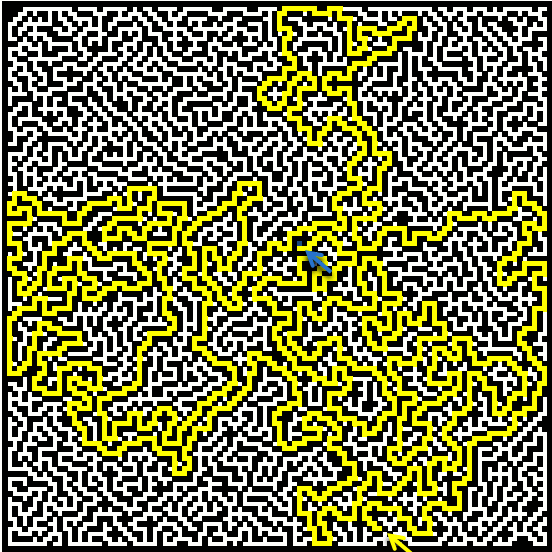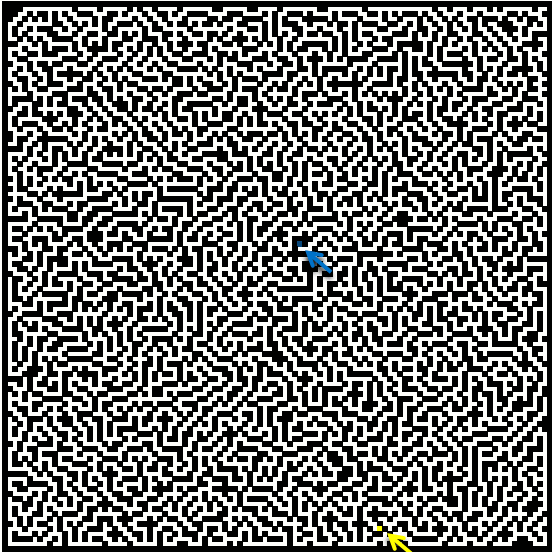Author: Oscar Cronquist Article last updated on November 12, 2020This article demonstrates a macro that finds a way between a start and an end point in a maze.  My last article showed you how to build a random maze programmatically. You might remember that there was only one path between the start point and the end point. The macro moves randomly around the maze and remembers where it has been.

As soon as it finds the end coordinates, it stops. Conditional formatting highlights the found path yellow. You can sometimes see the macro going back and trying a new path. I have inserted two arrows to make it easier for you to find the start point and the end point. The animated image below shows the macro highlighting a path between the start and end point.### Show visited cellsYou can change the macro to also show paths that it did search but couldn't find the end point. The image above shows that it has been almost all over the maze, in this particluar example.

It is also possible to highlight visited cells, change this line:

```Range("B2:DG111").Cells(loc(0, UBound(loc, 2)), loc(1, UBound(loc, 2))) = ""
```

to

```Range("B2:DG111").Cells(loc(0, UBound(loc, 2)), loc(1, UBound(loc, 2))) = "V"
```

Also create a new conditional formatting rule:

=B2="V"

Apply the rule to cell range =\$B\$2:\$DG\$111. I picked a grey formatting color.

### VBA Code

```'Name macro
Sub FindPath()
'Dimension variable and declare data type
Dim loc() As Variant

'Redimension variable loc to make it an array variable
ReDim loc(1, 0)

'Disable screen refresh
Application.ScreenUpdating = False

'Search for a cell matching text value "S" and save it to an object named Sp (Start point)
Set Sp = Cells.Find("S", LookIn:=xlValues, LookAt:=xlWhole, SearchOrder:=xlByRows, SearchDirection:=xlNext)

'Save the column number of the start cell to array variable loc position row 1 and column 0
loc(1, 0) = Sp.Column - 1

'Save the row number of the start cell to array variable loc position row 0 and column 0
loc(0, 0) = Sp.Row - 1

'Search for a cell matching text value "E" and save it to an object named Ep (End point)
Set Ep = Cells.Find("E", LookIn:=xlValues, LookAt:=xlWhole, SearchOrder:=xlByRows, SearchDirection:=xlNext)

'Save the column number of the end cell to variable Ec
Ec = Ep.Column - 1

'Save the row number of the end cell to variable Er
Er = Ep.Row - 1

'Save values in cell range B2:DG111 to variable mtx
mtx = Range("B2:DG111").Value

'Clear End point value in array variable mtx based on variables Er and Ec
mtx(Er, Ec) = ""

'Save 0 (zero) to variable Counter
Counter = 0

'Do ... Loop Until statement - Repeat executing lines between Loop and Loop Until until a given condition is met
Do

'Save boolean value False to variable chk
chk = False

'Check if a values in array variable mtx is equal to "" (nothing), if so change variable chk to boolean value True
'In other words check if a value next to the current location is equal to "" (nothing)
'Values next to the current location can be the adjacent value up, down, right or left.
If mtx(loc(0, UBound(loc, 2)) + 1, loc(1, UBound(loc, 2))) = "" Then chk = True
If mtx(loc(0, UBound(loc, 2)) - 1, loc(1, UBound(loc, 2))) = "" Then chk = True
If mtx(loc(0, UBound(loc, 2)), loc(1, UBound(loc, 2)) + 1) = "" Then chk = True
If mtx(loc(0, UBound(loc, 2)), loc(1, UBound(loc, 2)) - 1) = "" Then chk = True

'Check if variable chk is equal to boolean value False
If chk = False Then

'Check if variable loc (current location) is equal to the starting point
If loc(0, UBound(loc, 2)) = Sp.Row - 1 And loc(1, UBound(loc, 2)) = Sp.Column - 1 Then

'Continue here if variable loc (current location) is not equal to the starting point
Else

'Save "" (nothing) to cell in cell range B2:DG111 based on values in array variable loc
Range("B2:DG111").Cells(loc(0, UBound(loc, 2)), loc(1, UBound(loc, 2))) = ""

'Redimension array variable loc
ReDim Preserve loc(UBound(loc, 1), UBound(loc, 2) - 1)

'Check if variable Tcounter is smaller than variable Counter
If Tcounter < Counter Then

'Save value in variable Counter to variable Tcounter
Tcounter = Counter

'
Exr = loc(0, UBound(loc, 2) - 1)
Exc = loc(1, UBound(loc, 2) - 1)
End If

'Subtract variable Counter with 1
Counter = Counter - 1
End If

'Continue here if variable chk is not equal to boolean value False
Else

'Redimension variable loc
ReDim Preserve loc(UBound(loc, 1), UBound(loc, 2) + 1)

'Save boolean value False to variable c
c = False

'Do ... Loop Until statement
'Repeat executing lines between Do and Loop until variable c equals boolean value True
Do Until c = True

'Create a random whole number between 0 (zero) and 3 and save it to variable rrand
rrand = Int(Rnd * 4)

'Select Case statement, allows you to control which lines to be rund based on the outcome of the random value
Select Case rrand

'Go here if variable rrand is equal to 0 (zero)
Case 0

'Check if cell value to the right of the current cell is equal to "" (nothing)
If mtx(loc(0, UBound(loc, 2) - 1) + 1, loc(1, UBound(loc, 2) - 1)) = "" Then
loc(0, UBound(loc, 2)) = loc(0, UBound(loc, 2) - 1) + 1
loc(1, UBound(loc, 2)) = loc(1, UBound(loc, 2) - 1)

'Save boolean value True to variable c
c = True
End If

'Go here if variable rrand is equal to 1
Case 1

'Check if cell value to the left of the current cell is equal to "" (nothing)
If mtx(loc(0, UBound(loc, 2) - 1) - 1, loc(1, UBound(loc, 2) - 1)) = "" Then
loc(0, UBound(loc, 2)) = loc(0, UBound(loc, 2) - 1) - 1
loc(1, UBound(loc, 2)) = loc(1, UBound(loc, 2) - 1)

'Save boolean value True to variable c
c = True
End If

'Go here if variable rrand is equal to 2
Case 2

'Check if cell value above the current cell is equal to "" (nothing)
If mtx(loc(0, UBound(loc, 2) - 1), loc(1, UBound(loc, 2) - 1) + 1) = "" Then
loc(0, UBound(loc, 2)) = loc(0, UBound(loc, 2) - 1)
loc(1, UBound(loc, 2)) = loc(1, UBound(loc, 2) - 1) + 1

'Save boolean value True to variable c
c = True
End If

'Go here if variable rrand is equal to 3
Case 3

'Check if cell value below the current cell is equal to "" (nothing)
If mtx(loc(0, UBound(loc, 2) - 1), loc(1, UBound(loc, 2) - 1) - 1) = "" Then
loc(0, UBound(loc, 2)) = loc(0, UBound(loc, 2) - 1)
loc(1, UBound(loc, 2)) = loc(1, UBound(loc, 2) - 1) - 1

'Save boolean value True to variable c
c = True
End If
End Select
Loop

'Save text value "S" to a value in array variable mtx
mtx(loc(0, UBound(loc, 2)), loc(1, UBound(loc, 2))) = "S"

'Save text value "S" to a cell in cell range B2:DG111
Range("B2:DG111").Cells(loc(0, UBound(loc, 2)), loc(1, UBound(loc, 2))) = "S"

Counter = Counter + 1
End If

k = k + 1

'Show changes on screen every 500 steps based on variable k
If (k / 500) - Int(k / 500) = 0 Then
Application.ScreenUpdating = True
Application.ScreenUpdating = False
End If
Loop Until loc(0, UBound(loc, 2)) = Er And loc(1, UBound(loc, 2)) = Ec

'Save text value "E" to end point
Range("B2:DG111").Cells(Er, Ec) = "E"

'Show changes on screen
Application.ScreenUpdating = True
End Sub
```

### Get the Excel fileFind-path-in-maze.xlsm